# Triganometry, Graphs & Measurements

?
• Created by: Kathryn
• Created on: 10-05-14 08:41

## Pythagoras Theorem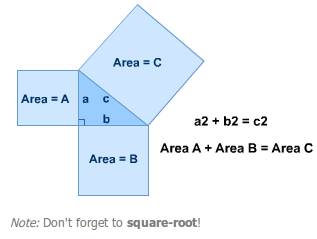1 of 15

## Sin, Cos, Tan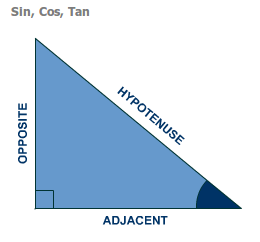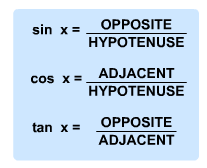2 of 15

## Formula Triangles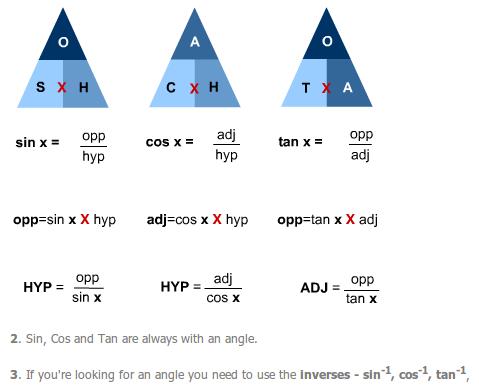3 of 15

## Linear Graphs

Linear functions can be written in the form y = mx + c where y and x are variables and m and c are constants (numbers).

If you write them like this then m is the gradient and c is the y-intercept (point where it crosses the y-axis). The graphs of linear functions are straight lines.

To find m:

Pick any two points.To find c:

c is the point where the graph crosses the y-axis.

4 of 15

Quadratic functions can be written in the form:

y = ax2 + bx + c

where a, b and c are constants and 'a' doesn't equal zero.

Quadratic graphs are always parabolas ('U' shapes).

The really important bits of a quadratic are:

Where it turns (the bottom of the 'U')

Where it crosses the x-axis (if it does!)

The solutions of a quadratic are where the graph crosses the x-axis!

5 of 15

## Cubics and Reciprocals

You need to be able to:

1. Plot and draw these.
2. Recognise the shapes.
3. Read the solutions from the graph (cubics only).

Cubics can be written in the form:

y = ax3 + bx2 + cx + d

Reciprocals are where the x is on the bottom of a fraction.

Drawing their graphs - Table - Axes - Plot - Draw - Label

The solutions of a cubic are where it crosses the x-axis and it can have up to 3.

6 of 15

## Graphs of simultaneous equations

As simultaneous equations at GCSE are linear (can both eb written in the form y = mx + c) their graphs will be straight lines.

The solution (x-value and y-value) is where the straight lines intersect (cross one another).

## Inequalities - regions on a graph

To draw a graph:

1. Change the inequality sign to an '=' sign.
2. By choosing 4 or 5 different values for x, make a table of co-ordinates.
3. Draw and label the line (make it dotted if the inequality sign is < or >).
4. Choose a test point (not on the line!).
5. Put the x and y values of the test point into the inequality.
6. If it works, shade and label that side of the line with the inequality.
7. If it doesn't work, shade and label the other side.
7 of 15

## Travel Graphs

Distance/time

If you show a graph of a journey showing distance travelled (on the y-axis) against time (on the x-axis):

• The gradient (or slope) of the graph represents the speed.
• A horizontal section indicates that you have stopped.
• A section sloping up means that you are going away.
• A section sloping down means you are coming back.
• The steeper the line, the faster you are going.

Speed/time

• The gradient (or slope) of the graph represents the acceleration.
• The area under the graph (for any section) is the distance travelled (in that section).
• A horizontal section indicates constant speed (no acceleration).
• A section sloping up means accelerating.
• A section sloping down means slowing down.
• The steeper the line, the quicker the acceleration.
8 of 15

## Area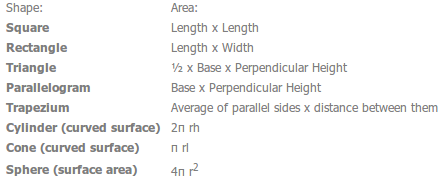9 of 15

## Volume10 of 15

## Metric

Length

1cm = 10mm

1m = 100cm

1km = 1000m

Mass:

1kg = 1000g

1 tonne = 1000kg

Volume:

1 litre = 1000ml = 1000cm3

Note to remember:

1m = 100cm 1m2 = 10 000cm2 1m3 = 1 000 000cm3

11 of 15

## Imperial

Here are some more facts that you should know:

Length:

1 foot = 12 inches

1 yard = 3 feet

1 mile = 1760 yards

Length:

1 pound = 16 ounces

1 stone = 14 pounds

1 ton = 160 stones (or 2240 pounds)

Note: the different spellings of tonne (metric) and ton (imperial).

Volume: 1 gallon = 8 pints

12 of 15

13 of 15

## Lotus

locus is simply a set of points that satisfy some sort of condition.

Distance from a point

A circle around the point!

Distance from two points

A perpendicular line straight down the middle of the points:

1. Set your compasses so that they are roughly the same as the distance between the points (or less if you don't have a lot of room!).
2. Put the point of the compasses on the first cross and do two arcs - one above the points and one below.
3. Put the point on the second cross and do the same thing so that you cross the first arcs (making sure you keep the compasses the same distance apart).
4. Now simply draw a line straight down the middle through the points where the arcs cross.
14 of 15

## Lotus (2)

Distance from two lines

The set of points that are the same distance from two lines is a straight line down the middle which bisects the angle (cuts it in half):

1. Get a pair of compasses and place the point where the two lines meet. Draw little arcs that cross each of the lines.
2. Now, keeping the compasses set, put the point on each line where your arc has crossed it and draw another little arc in-between the two lines. You should now have another two little arcs in the middle.
3. Draw a straight line from the angle through the point where your little arcs cross and you've done it!
15 of 15